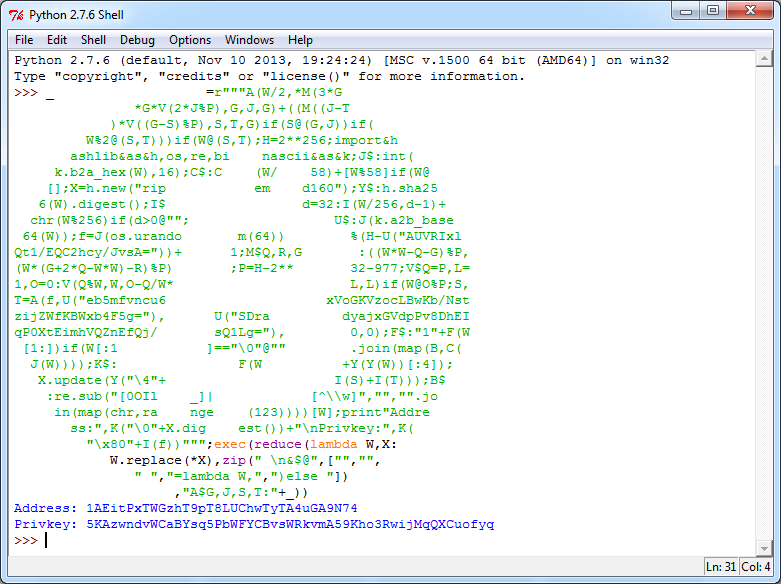Bitcoin Address Generator in Obfuscated Python

# Bitcoin Address Generator in Obfuscated Python

##### May 19, 2019

Never underestimate what programmers can do.

The code below shows a fully-functioning Bitcoin address generator in obfuscated Python (2.5-2.7), which I saw in an interesting article posted in 2013.

_                   =r"""A(W/2,*M(3*G
*G*V(2*J%P),G,J,G)+((M((J-T
)*V((G-S)%P),S,T,G)if(S@(G,J))if(
W%2@(S,T)))if(W@(S,T);H=2**256;import&h
ashlib&as&h,os,re,bi    nascii&as&k;J$:int( k.b2a_hex(W),16);C$:C    (W/    58)+[W%58]if(W@
[];X=h.new("rip           em    d160");Y$:h.sha25 6(W).digest();I$                 d=32:I(W/256,d-1)+
chr(W%256)if(d>0@"";                  U$:J(k.a2b_base 64(W));f=J(os.urando m(64)) %(H-U("AUVRIxl Qt1/EQC2hcy/JvsA="))+ 1;M$Q,R,G       :((W*W-Q-G)%P,
(W*(G+2*Q-W*W)-R)%P)       ;P=H-2**       32-977;V$Q=P,L= 1,O=0:V(Q%W,W,O-Q/W* L,L)if(W@O%P;S, T=A(f,U("eb5mfvncu6 xVoGKVzocLBwKb/Nst zijZWfKBWxb4F5g="), U("SDra dyajxGVdpPv8DhEI qP0XtEimhVQZnEfQj/ sQ1Lg="), 0,0);F$:"1"+F(W
[1:])if(W[:1           ]=="\0"@""        .join(map(B,C(
J(W))));K$: F(W +Y(Y(W))[:4]); X.update(Y("\4"+ I(S)+I(T)));B$
:re.sub("[0OIl    _]|            [^\\w]","","".jo
W.replace(*X),zip(" \n&$@",["","", " ","=lambda W,",")else "]) ,"A$G,J,S,T:"+_))